error: Content is protected !!

# MATHEMATICS: FORM THREE: Topic 2 - FUNCTIONS

TOPIC 2: FUNCTIONS
Normally relation deals with matching of elements from the first set called DOMAIN with the element of the second set called RANGE.
Definitions:
A function is a relation with a property that for each element in the domain there is only one corresponding element in the range or co- domain
Therefore functions are relations but not all relations are functions
The Concept of a Functions Pictorially
Explain the concept of a functions pictorially
Example 1
Which of the following relation are functions?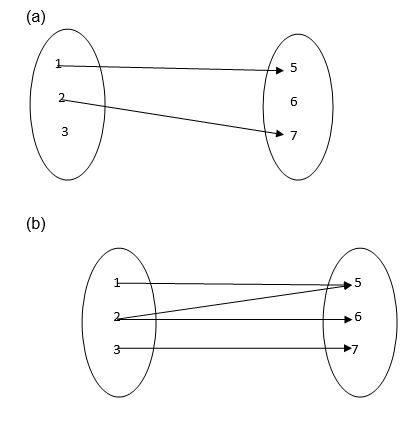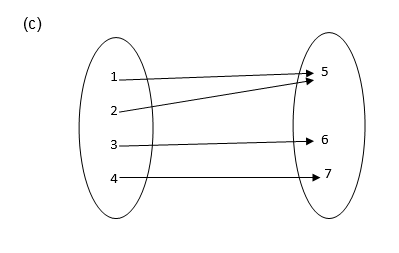Solution
1. It is not a function since 3 and 6 remain unmapped.
2. It is not a function because 2 has two images ( 5 and 6)
3. It is a function because each of 1, 2, 3 and 4 is connected to exactly one of 5, 6 or 7.
Functions
Identify functions
TESTING FOR FUNCTIONS:
If a line parallel to the y-axis is drawn and it passes through two or more points on the graph of the relation then the relation is not a function.
If it passes through only one point then the relation is a function
Example 2
Identify each of the following graphs as functions or not.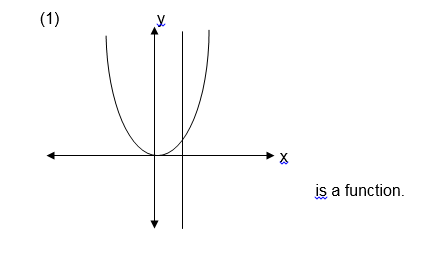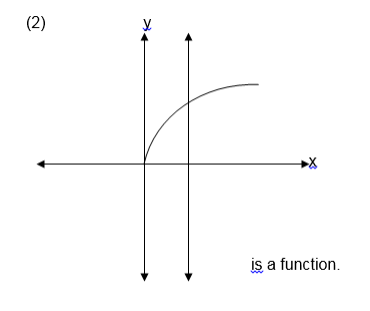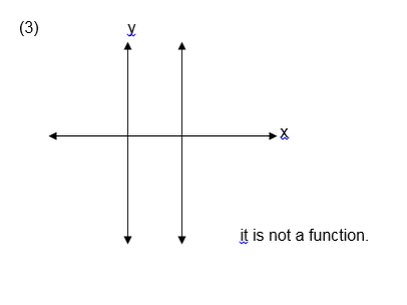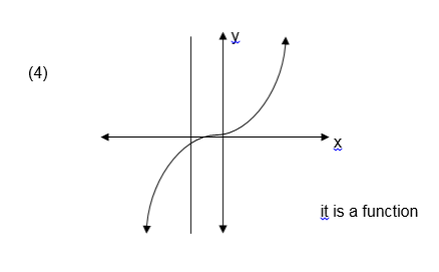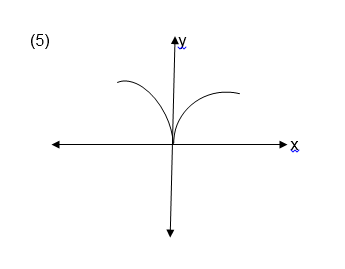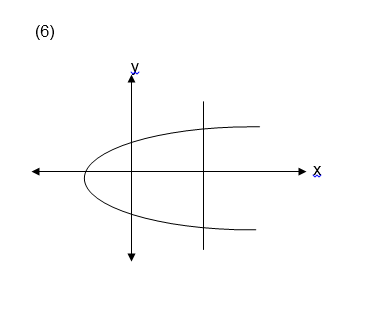Exercise 1
1. Which of the following relations are functions?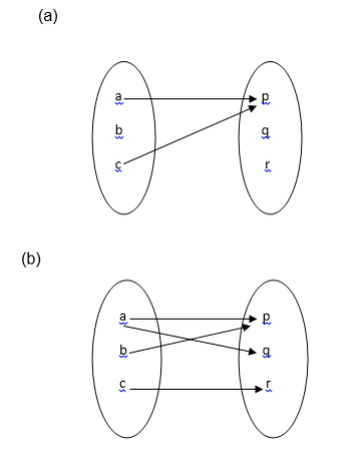2. Let A = {1, 2, 3, 4, 5, 6, 7, 8, 9, 10}
and B ={ 2, 3, 5, 7 }
Draw an arrow diagram to illustrate the relation “ is a multiple of ‘ is it a function ? why?
3. let A = {1,-1 ,2,-2} and
B = {1, 2, 3, 4 } which of the following relations are functions ?
1. { ( x , y ) : x < y }
2. { ( x , y ) : x > y}
3. { ( x , y ) : y = x2}

The Domain of a Function
State the domain of a function
If y = f (x),that is y is a function of x ,then domain is a set of x values that satisfy the equation y = f (x).
The Range of Function
State the range of function
If y = f (x),that is y is a function of x , then therange is a set of y value satisfying the equation y = f (x).
Example 3
1. Let f(x) = 3x – 5 for all value of x such that -2 £ x £ 3 find its range
Solution
f (x) = y = 3x -5
When x = -2
f(-2) = y = 3x(-2)-5 = -11 , so (x,y)=(-2,-11)
f(3) =y= 3x3-5 = 4, so when x = 3 , y = 4
Therefore y is found in between – 11 and 4
Range ={ y: - 11≤ y≤ 4}
Example 4
If f (x) = x– 3, state the domain and range of f (x)
Solutions;
Domain = all real numbers
Range:
f(x) = y = x2 – 3
Make x the subject
y+ 3 = x2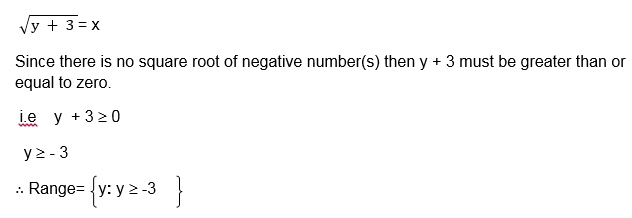Exercise 2
1. For each of the following functions, state the domain and range
1. f(x) = 2x + 7 for 2 £ x £ 5
2. f(x) = x – 1 for -4 £ x £ 6
3. f(x) = 5 - 3x such that -2 £ f(x) < 8
2. for each of the following functions state the domain and range
1. f(x) = x2
2. f(x) = x2+2
3. f(x) = 2x + 1
4. f(x) = 1 – x2
Exercise 3
1.The range of the function
f(x) 3 – 2x for 0 ³0 x £7 is;
1. y: -18£ y £3
2. y: -3£ y £18
3. y: 3 £y £18
4. y: -18 £ y £-3
2. The range of the function
f(x)=2x+1 is y: -3£ y £17 what is the domain of this function?
1. x: - 3£ x £17
2. x: - 2£ x £8
3. x: -17 £ x £3
3.Which of the following relations represents a function:
1. R = (x, y) : y = for x ≥0
2. R= (x, y) : y= x-2 for x ≥0
3. R= (x, y) : y = for x ≥0 and y ≥0
4. R= (x, y) : x = 7 for all values of y
4.Which of the following relations is a function:
1. R = (x, y): -2 £ x £6, 3 £ y<8 and x<y, Where both x and y are integers
2. R= (x, y): -2 £ x £6, 3 £ y<8 and x<y, Where both x and y are integers
3. R= (x,y): y = √(x+2) for x ≥-2.
4. R = (x, y): y=√(2-x) for x ≤2 and y ≤0
5.Let f (x) = x+ 1. Which of the following is true?
1. f (-2) < f (0)
2. f (3)> f (-4)
3. f (-5) = f (5)
4. The function crosses , y – axis at 1

One to one and many to one functions:
One to functions;
A one to one function is a function in which one element from the domain is mapped to exactly one element in the range:
That is if a ≠b then f (a) ≠f (b)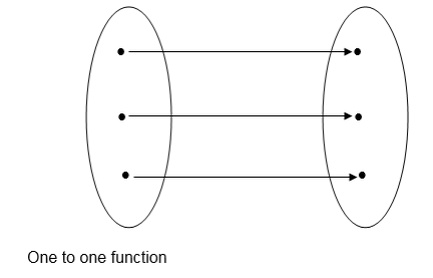Many to one function;
This is another type of function with a property that two or more elements from the domain can have one image (the same image).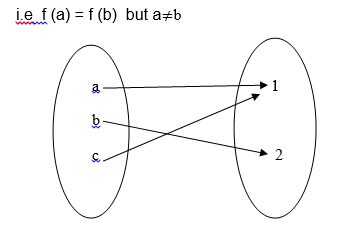Examples of one to one functions
1. f (x) = 3x + 2
2. f (x) = x + 6
3. f (x) = x3 + 1 etc
Examples of many to one function
1. f(x) = x2 +1
2. f(x) = x4 – 2 etc
NB. All functions with odd degrees are one to one function and all functions with even degrees are many to one functions.
Example 5
Let A = -2, -1, 0, 1, 2 and B = 0, 1, 4 and the function f mapping each element from set A to those of B is defined as f(x)=x2.Is f one to one function?Example 6
Let P = {-2, -1, 0,1,2} and
Q = {-1, 0, 1, 2, 3}
g(x) = x + 1, is g one to one function?
Solution: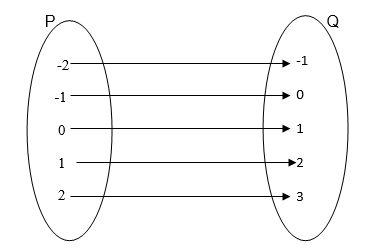g (x) is one to one function because every element in P has only one image in Q
NB: In example 1, f(x) is not a one to one function because -2 and 2 in A have the same image in B, that is 4 is the image of both 2 and -2.
Also 1 is the image of both 1 nd -1.
Example 7
State whether or not if the following graphs represent a one to one function: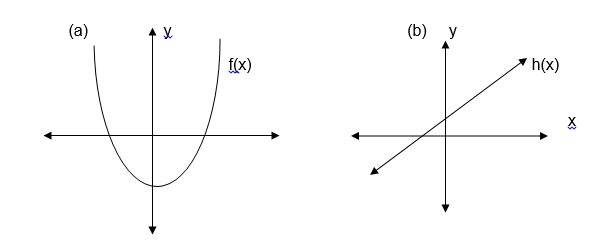Solution:
Draw a line parallel to the x axis and see if it crosses the graph at more than one points. If it does, then, the function is many to one and if it crosses at only one point then the graph represents a one to one function.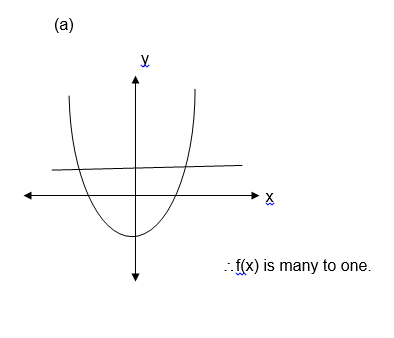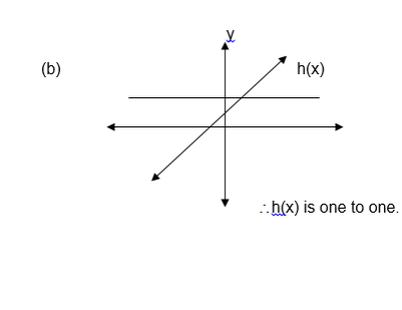Graphs of Functions
Draw graphs of functions
Many functions are given as equations, this being the case, drawing a graph of the equation is obtaining the graph of the equation which defines the function.
Note that, you can draw a graph of a function if you know the limits of its independent variables as well as dependent variables. i.e you must know the domain and range of the given function.
Example 8
Draw the graph of the following functions
1. f(x) = 3x -1
2. g (x) = x2 – 2x -1
3. h (x) = x3
Solution
f(x) = 3x – 1
The domain and range of f are the sets of all real numbers
f(x) = y = 3x – 1
So y = 3x – 1
Table of value :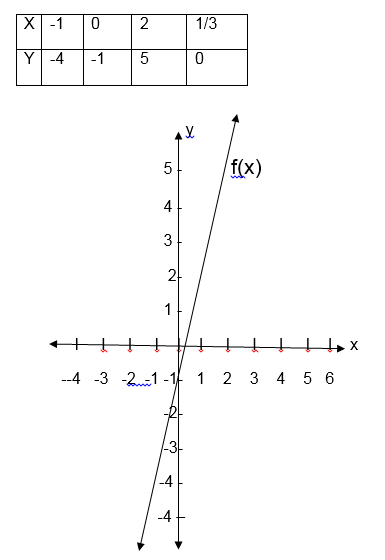g(x) = x2 -2x -1
y=x2-2-1
a=-1, b=-2 1 and c=-1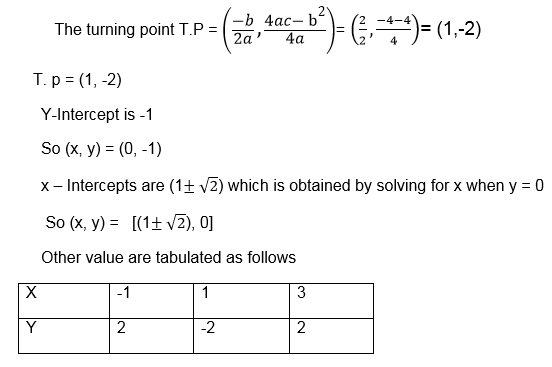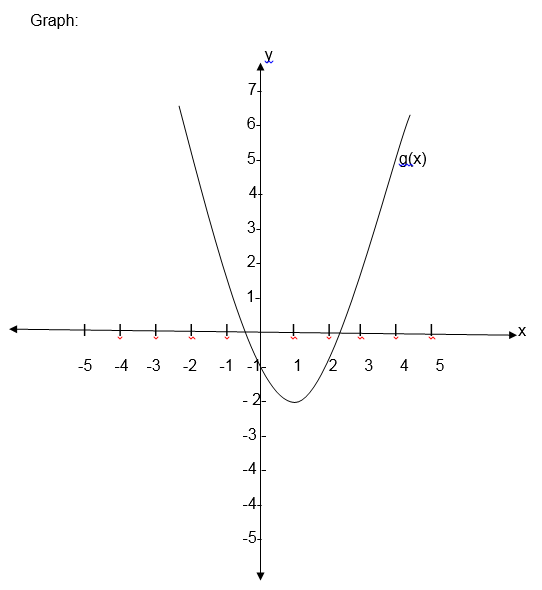forh(x) = x3
Solution: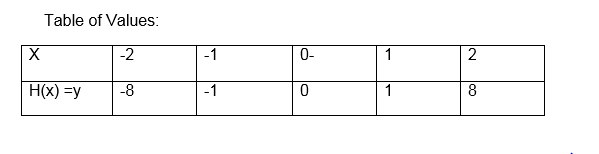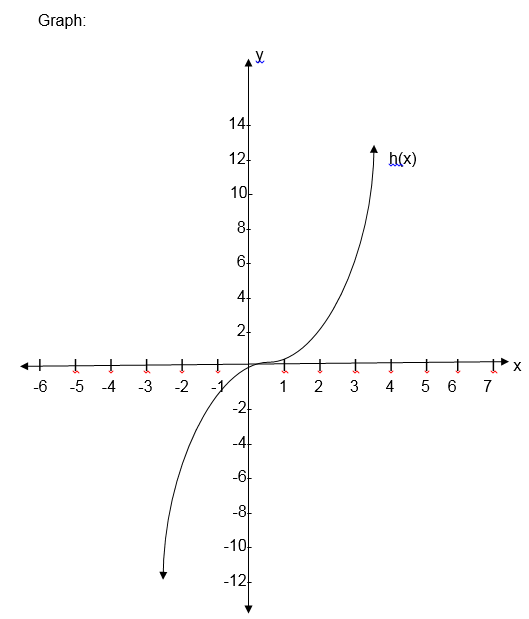The first graph is the graph of linear function, the second one is called the graph of a quadratic function and the last graph is for cubic function.
Example 9
Draw a graph of the function:
f(x) = -1 + 6x-x2
Solution:
a=-1, b=6, c=-1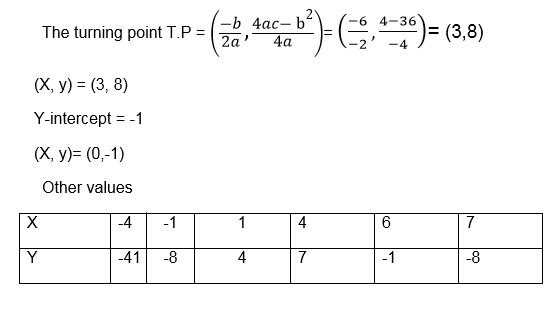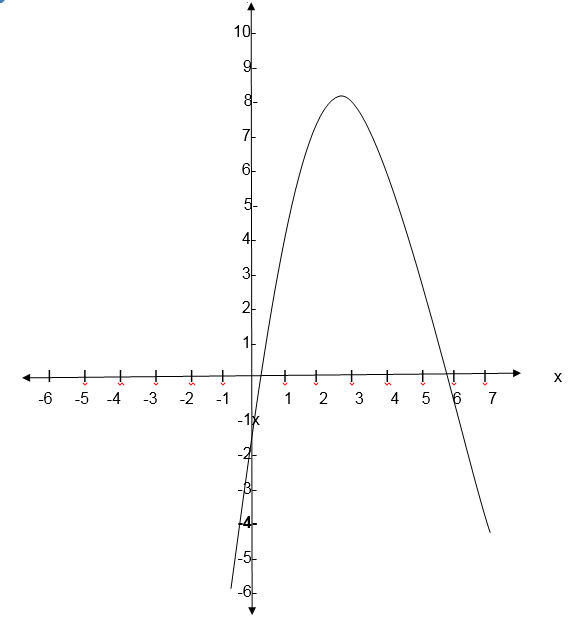Exercise 4
1.Which of the following are one to one function?
1. f(x) = 3x – x2
2. g (x) = x-1
3. k(x) =x3+1
4. f(x) =x+x2+x3
5. k(x)=x4
2. Draw the graph of the following functions:
1. f(x) = 3x – x2
2. h (x) = x+1
3. g(x) =x 3- x 2+3
3. At what values of x does the graph of the function f(x) = x2+x-6 cross thex- axis?
1. x=-3 and x=7
2. x=8 and x=-6
3. x=-3 and x=2
4. x=4 and x=-1
4. Which of the following function is one to one function?
1. f(x)=x2+2
2. f(x) =x4-x2
3. f(x)=x5-7
4. f(x)=x2+x+2

Functions with more than one part.
Some functions consist of more than one part. When drawing their graphs draw the parts separately.
If the graph includes an end point, indicate it with a solid dot if it does not include the end point indicate it with a hollow dot.
E.g. draw the graphs of the functions
(a) F(x) x+1 for x>0
(b) f(x)=x+1for x³0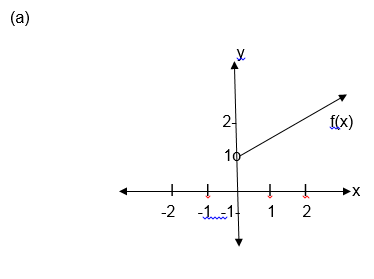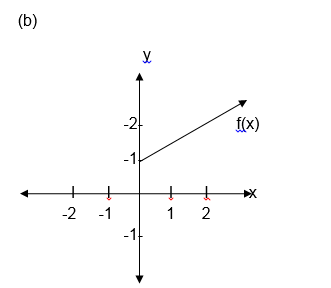Example 10
Step function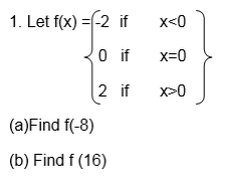(c) Sketch its graph
(d) State the domain and range of f
Solution: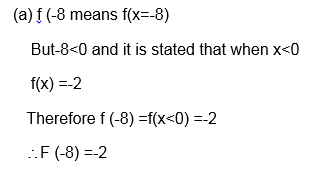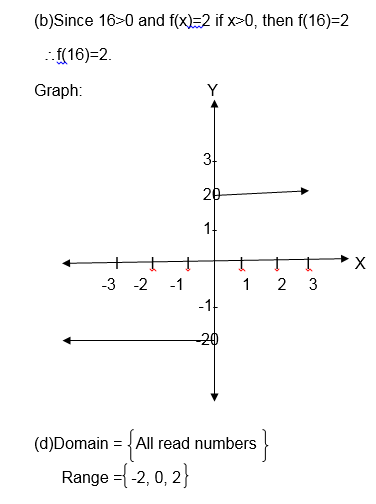Exercise 5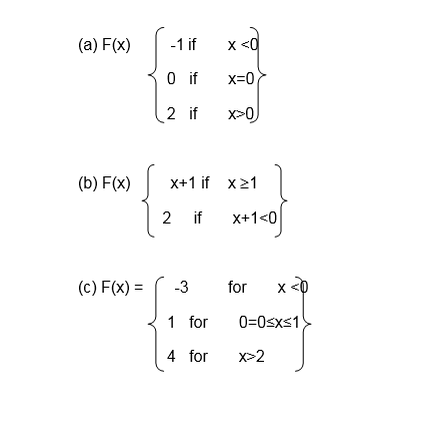Absolute value functions (Modulus functions)
The absolute function is defined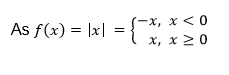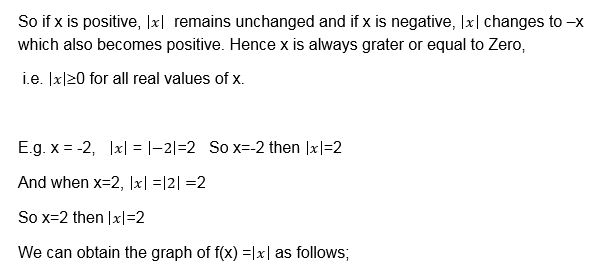Table of values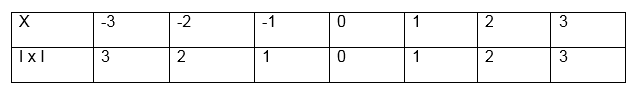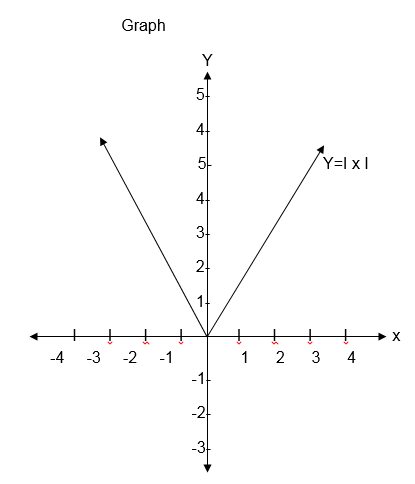Example 11
Solve the following: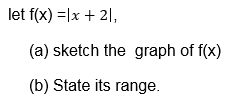Solution
table of values.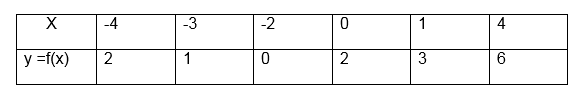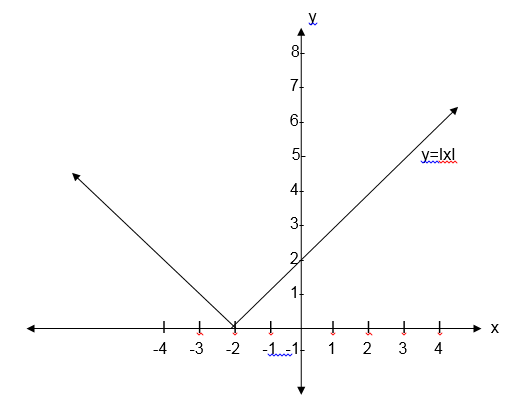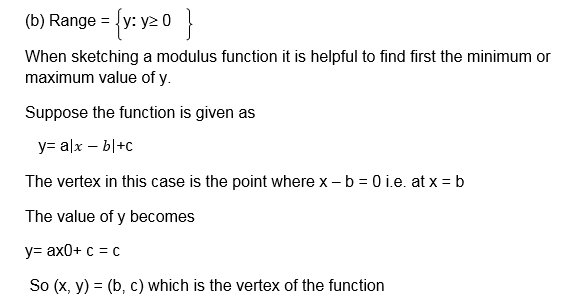Step functions: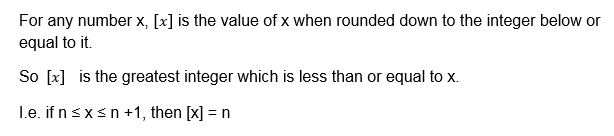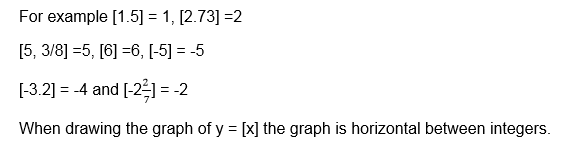Example 12
Draw the graph of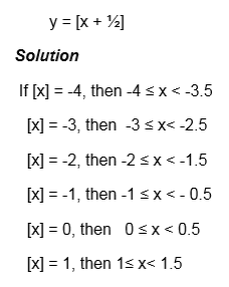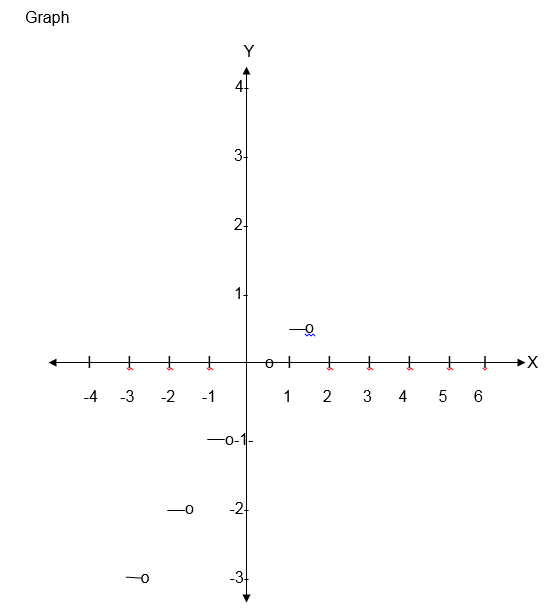Note that the graph obtained is like steps such functions are called steps functions
Exercise 6
1. Draw the graph of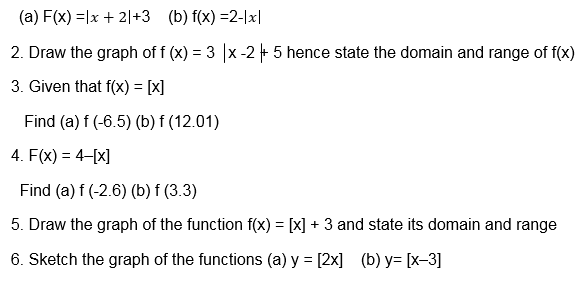The Inverse of a Function
Explain the inverse of a function
In the discussion about relation we defined the inverse of relation.
It is true that the inverse of the relation is also a relation.
Similarly because a function is also relation then every function has its inverse
The Inverse of a Function Pictorially
Show the inverse of a function pictorially
According to the definition of function the inverse of a function is also a function if and only if the function is one to one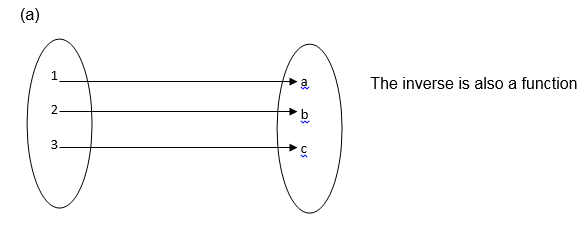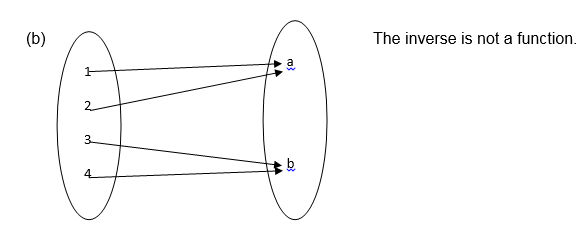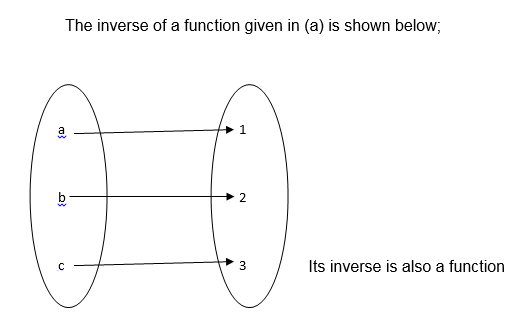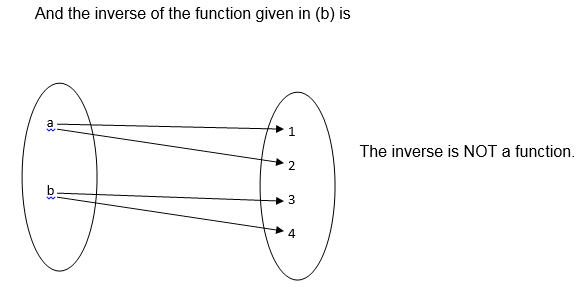The Inverse of a Function
Find the inverse of a function
If the function f is one to one function given by an equation, then its inverse is denoted by f-1 which is obtained by inter changing the variables x and y then making y the subject of the formula.
I.e. If y=f(x), then x = f-1 (y)
Example 13
1. Find the inverse of each of the following functions;
1. F(x) = 3x-6
2. F(x) =x3
Solution: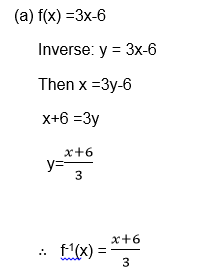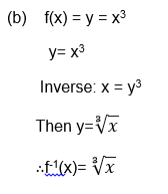A Graph of the Inverse of a Function
Draw a graph of the inverse of a function
Example 14
find the inverse of the function f(x) = x-5 and then sketch the graph of f-1(x) , also state the domain and range of f-1(x).
solution: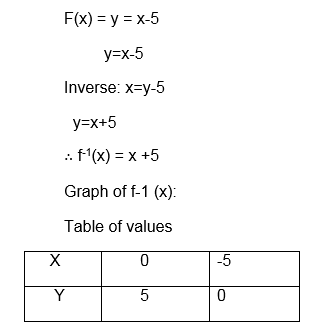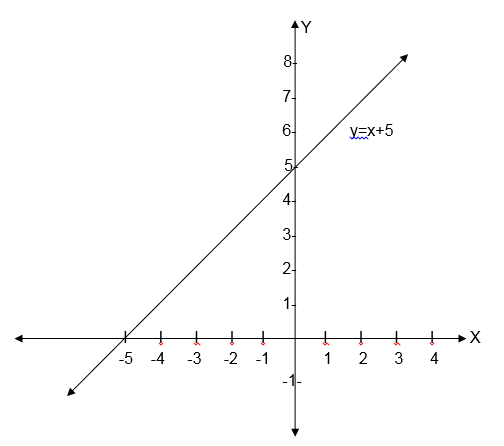Domain = {All real numbers}
Range = {All real numbers}
NB: if a function f takes a domain A to a range B, then the inverse f-1 takes B back to A.
Hence the domain of f-1 is the range of f, and the range of f-1 is the domain of f.
The Domain and Range of Inverse of Functions
State the domain and range of inverse of functions
Example 15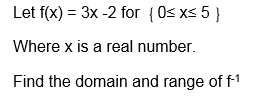Solutions: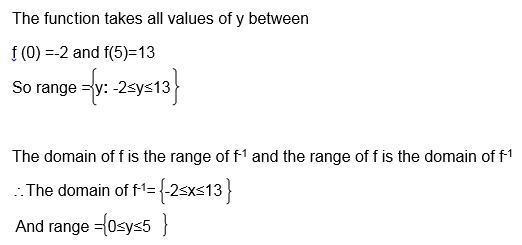1. Given that f(x) = x2-2[x] +3, what is the value of f (-4)?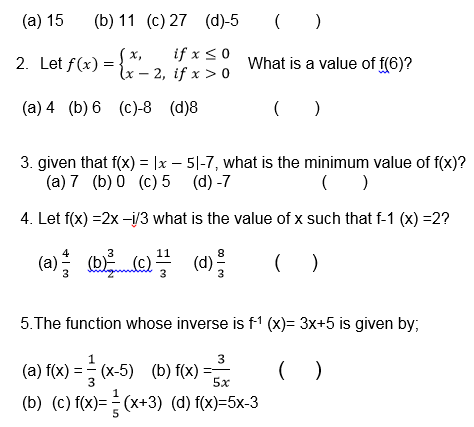1.2.